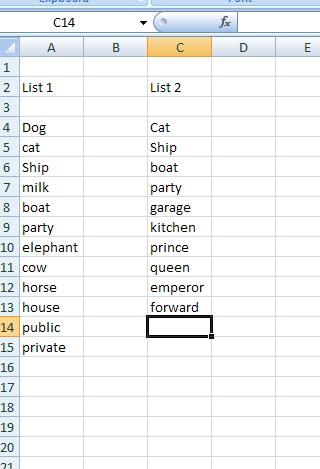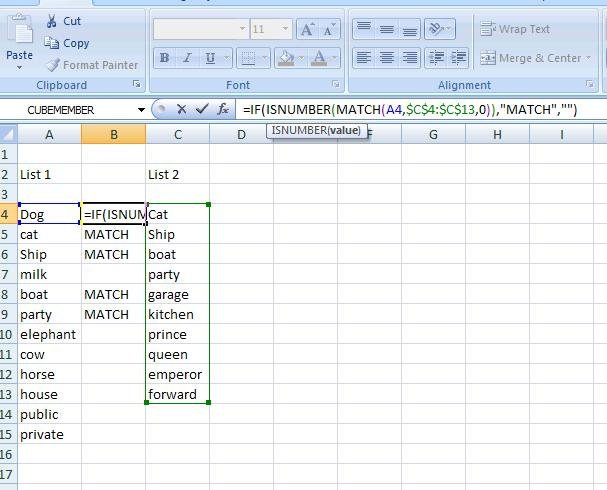# Comparing Items in a List

Consider the following two lists:And that we want to see which items from column A are in Column B. This can be achieved by the MATCH function in conjunction with ISNUMBER .

The MATCH function has the following syntax:

MATCH(A6,C4:C13,0)

And this will return the position of the value cell A6 in the range C4:C13 – the “0” is for an exact match. So this will return the number 2 – as “Ship” (the value in cell A6) has position 2 in the range C4:C13. If an exact match can’t be found then an N/A# is returned.

Combining this with a ISNUMBER function we have:

ISNUMBER(MATCH(A6,C4:C13,0))

Will return TRUE when there is a MATCH and a FALSE when there is no MATCH.

So we can put the following in cell B4 and copy down:

IF(ISNUMBER(MATCH(A4\$C\$4:\$C\$13,0)),”MATCH”,””)

This will return the expression “MATCH” when the value in Column A can be found in Column B